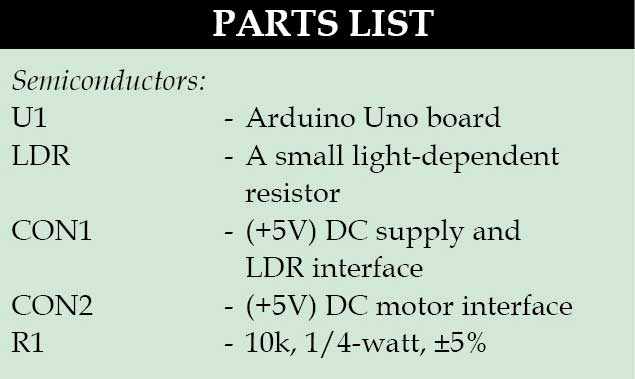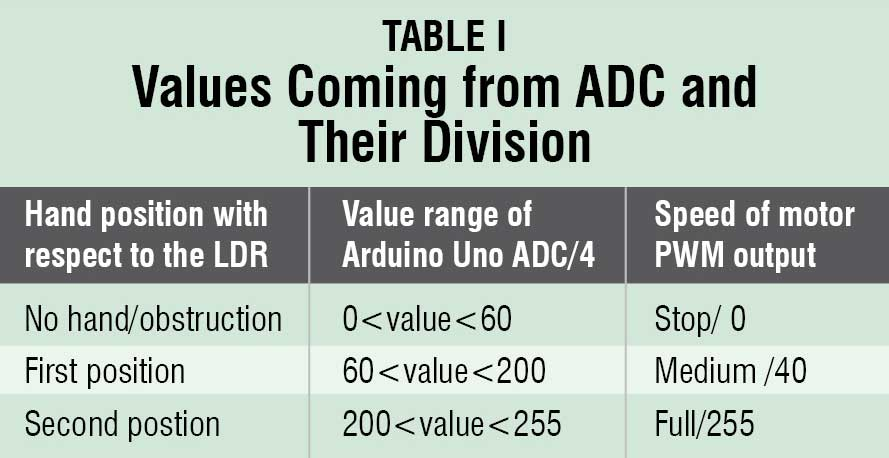A light-dependent resistor (LDR) whose resistance is inversely proportional to the intensity of light is often used as a sensor in electronic projects that involve the use of light. This LDR based DC motor speed control project uses an LDR to control the speed of a DC motor.The objective of this project is to see how much faster the motor moves when a hand is brought closer to it. This circuit could be very useful in applications where one tries to control the movement of a robot using a wired/wireless channel, keeping different arrangements of LDR and transmitting the same through the channel to the receiving side, where the movement of the robot can be controlled. It has been developed and tested keeping this vision in mind.

## DC motor speed control circuit

The circuit uses a small LDR, which is sensitive to the intensity of light. Voltages generated from the LDR are compared using an internal ADC of Arduino Uno, which turns on as light intensity goes above a certain voltage threshold. This voltage has already been calibrated for specific positions of the hand with respect to the LDR.In this project three variations in speeds are considered: stop, medium and high. Readings from the LDR are taken by analogue pin A0 using a voltage divider circuit consisting of 10k resistance and LDR connected at CON1 as shown.

Arduino Uno has a 10-bit ADC and its value ranges from zero to 1024. But, the pulse-width modulation (PWM) output from Arduino ranges from zero to 255. Thus, values coming from the ADC decrease by four times. These are divided into three ranges as shown in Table I.[stextbox id=”grey” caption=”Software programme”]

if(val<60) analogWrite(9,0); If 60>LDR output, send PWM (0) value to pin 9 of Arduino Uno.

if(val>60 && val<200) analogWrite(9,40); If 60 if(val>200 && val<255)
analogWrite(9,255);

If 200[/stextbox]

EFY note. LDR based speed variation is a very easy way of varying the speed of a DC motor. Change in speed from one to another is very smooth. But the number of distinct variations in speed is less. Only three prominent variations in 5V could be observed.

1.prasath

what is tp0 and tp1 in the circuit diagram..please explain

2.Rahul

3.Jazz
•EFY Team
4.Raghunathan